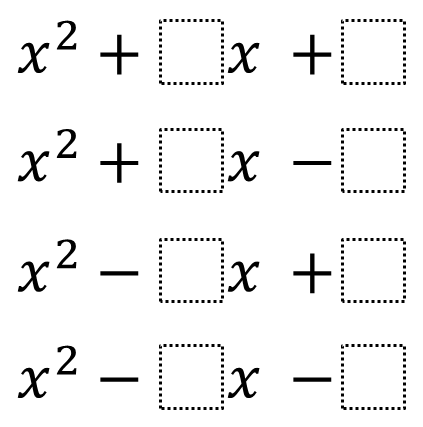Directions: Using the digits 1 to 9 at most one time each, place a digit in each box to create four different quadratic expressions that can be factored as two binomials with integer coefficients and terms.### Hint

(Note the changes in sign).
Try starting with a random c (constant term), then determine b (first-degree term).

x^2+6x+9
x^2-2x+1
x^2+4x-5
x^2-7x-8
(Others are possible)

Source: Giles Fox